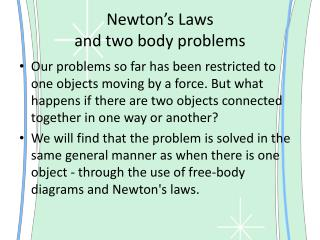DownloadDownload PresentationNewton’s Laws and two body problems

# Newton’s Laws and two body problems

Download Presentation## Newton’s Laws and two body problems

- - - - - - - - - - - - - - - - - - - - - - - - - - - E N D - - - - - - - - - - - - - - - - - - - - - - - - - - -
##### Presentation Transcript

1. Newton’s Laws and two body problems • Our problems so far has been restricted to one objects moving by a force. But what happens if there are two objects connected together in one way or another? • We will find that the problem is solved in the same general manner as when there is one object - through the use of free-body diagrams and Newton's laws.

2. Example problem 1: • A 5.0-kg and a 10.0-kg box are touching each other. A 45.0-N horizontal force is applied to the 5.0-kg box in order to accelerate both boxes across the floor. Ignore friction and determine the acceleration of the boxes and the force acting between the boxes. (Contact Force)

3. Note that there are four forces on the 5.0-kg object at the rear. • Using Fnet = m•a with the free-body diagram for the 5.0-kg object will yield the Equation below: • 45.0 - Fcontact = 5.0•a • Using Fnet = m•a with the free-body diagram for the 10.0-kg object will yield the Equation below: • Fcontact = 10.0•a

4. If the expression 10.0•a is substituted into Equation 1 for Fcontact, then Equation 1 becomes reduced to a single equation with a single unknown. The equation becomes • 45.0 - 10.0•a = 5.0•a • A couple of steps of algebra lead to an acceleration value of 3.0 m/s2

5. This value of a can be substituted back into Equation 2 in order to determine the contact force: • Fcontact = 10.0•a = 10.0 •3.0Fcontact = 30.0 N

6. Example Problem 2: • A man enters an elevator holding two boxes - one on top of the other. The top box has a mass of 6.0 kg and the bottom box has a mass of 8.0 kg. The man sets the two boxes on a metric scale sitting on the floor. When accelerating upward from rest, the man observes that the scale reads a value of 166 N; this is the upward force upon the bottom box. Determine the acceleration of the elevator (and boxes) and determine the forces acting between the boxes.

7. The force of gravity is calculated in the usual manner using 14.0 kg as the mass. • Fgrav = m•g = 14.0 kg • 9.8 N/kg = 137.2 N • Since there is a vertical acceleration, the vertical forces will not be balanced; the Fgrav is not equal to the Fnorm value. • The net force is the vector sum of these two forces. So • Fnet = 166 N, up + 137.2 N, down = 28.8 N, up

8. The acceleration can be calculated using Newton's second law: • a = Fnet /m = 28.8 N/14.0 kg = 2.0571 m/s2 • Now that acceleration has been determined, using an individual object, either box, we can determine the force acting between them. • Fcontact - 58.8 N = (6.0 kg)•(2.0571 m/s2)

9. Pulley Problems (omg) • 1. Consider the two-body situation below. A 100.0-gram hanging mass (m2) is attached to a 325.0-gram mass (m1) at rest on the table. The coefficient of friction between the 325.0-gram mass and the table is 0.215. Determine the acceleration of the system and the tension in the string.

10. a = 0.695 m/s2 and Ftens = 0.911 N • The solution here will use the approach of a free-body diagram and Newton's second law analysis of each individual mass.

11. For mass m1:Fgrav = m1•g = (0.3250 kg)•(9.8 N/kg) = 3.185 NFnorm = Fgrav = 3.185 NFfrict = µ•Fnorm = (.215)•(3.185 N) = 0.68478 N • Applying Newton's second law to m1:Ftens - 0.68478 = 0.3250•a • For mass m2:Fgrav = m2•g = (0.1000 kg)•(9.8 N/kg) = 0.9800 N • Applying Newton's second law to m2:0.9800 - Ftens = 0.1000•a

12. Combining the two equations leads to the answers:a = 0.69464 m/s2 = ~0.695 m/s2Ftens = 0.91054 N = ~0.911 N

13. Example 2 Pulley • Consider the two-body situation at the right. A 3.50x103-kg crate (m1) rests on an inclined plane and is connected by a cable to a 1.00x103-kg mass (m2). This second mass (m2) is suspended over a pulley. The incline angle is 30.0° and the surface has a coefficient of friction of 0.210. Determine the acceleration of the system and the tension in the cable.

14. Because the parallel component of gravity on m1 exceeds the sum of the force of gravity on m2 and the force of friction, the mass on the inclined plane (m1) will accelerate down it and the hanging mass (m2) will accelerate upward. • For mass m1:Fgrav = m1•g = (3500 kg)•(9.8 N/kg) = 34300 NFparallel = m1•g•sine(θ) = 34300 N • sine(30°) = 17150 NFperpendicular = m1•g•cosine(θ) = 34300 N • cosine(30°) = 29704.67 NFnorm = Fperpendicular = 29704.67 NFfrict = µ•Fnorm = (.210)•(29704.67 N) = 6237.98 N

15. Applying Newton's second law to m1:17150 N - 6237.98 N - Ftens = (3500 kg)•a • For mass m2:Fgrav = m2•g = (1000 kg)•(9.8 N/kg) = 9800 N • Applying Newton's second law to m2:Ftens - 9800 N - = (1000 kg)•a • Combining the two equations leads to the answers:a = 0.24712 m/s2 = ~0.247 m/s2Ftens = 10047 N = ~1.00x104 N